# Finding frequency of a specific mechanical oscillator -- horizontal rod on pivot

srecko97

## Homework Statement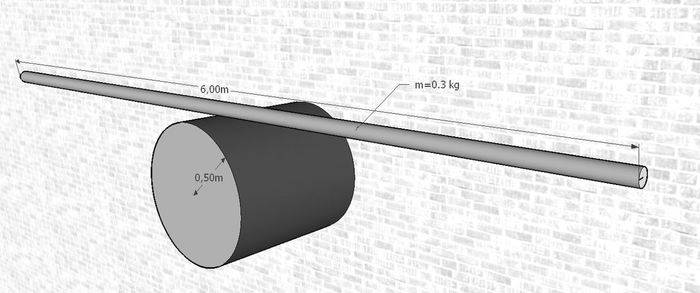There is a cyllinder with radius 0.5 m fixed on the wall. We put a 6 metres long thin rod with mass 0.3 kg on it, which does not slip. I would like to calculate the oscillating time. It is a part of a clock, so the oscillating time is probably 1 or 2 seconds, but I got around 5 seconds. Please help me find a mistake.

## Homework Equations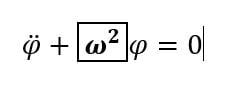## The Attempt at a Solution

I got a wrong result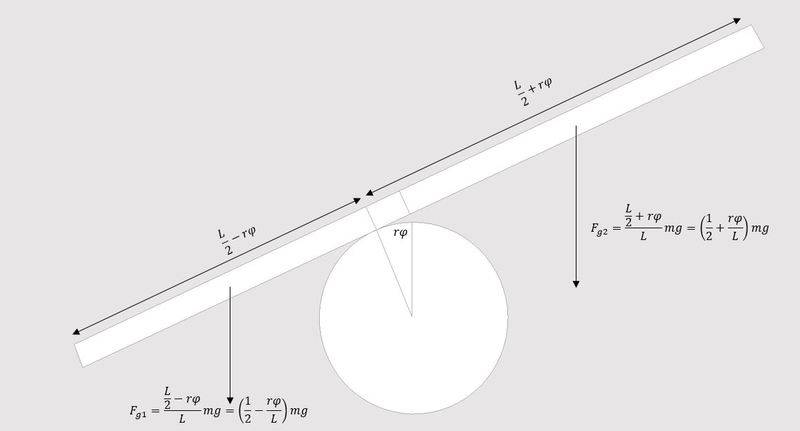I am used to write M (torque) and J (moment of inertia)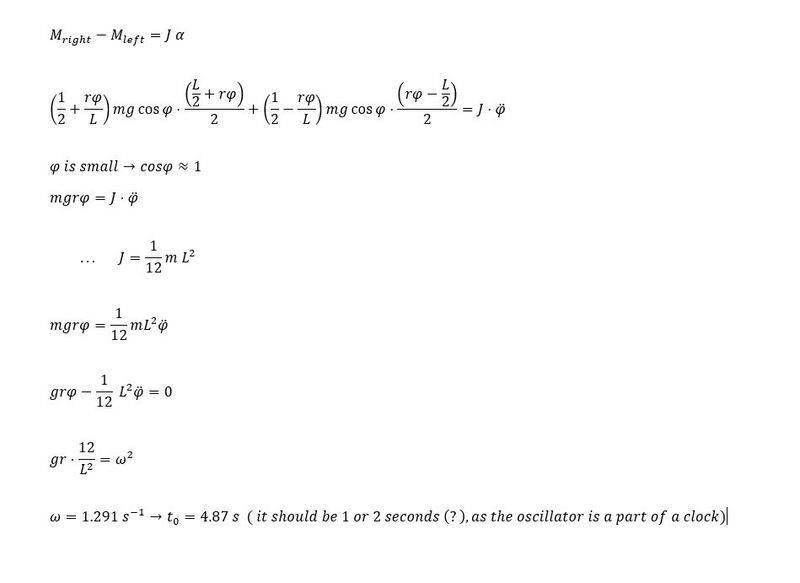[/B]

Last edited:

## Answers and Replies

Homework Helper
Gold Member
Your work looks correct. As a check, I used a different approach and still got the same answer.

•berkeman and srecko97
srecko97
Your work looks correct. As a check, I used a different approach and still got the same answer.
Thanks for your reply ... I will wait for my schoolmates to solve it in order to compare our results. I am quite sure that everything is correct except the calculation of moment of inertia. Should I treat each part of the rod separately (the longest and the shortest part using a formula for a rod rotating around one end .. (mx^2)/3) and sum moments of inertia of both parts as it does not oscillate accurately around its centre of mass.
I still think that the answer is not correct, so everyone, please help me solve it.

Homework Helper
Gold Member
Thanks for your reply ... I will wait for my schoolmates to solve it in order to compare our results. I am quite sure that everything is correct except the calculation of moment of inertia. Should I treat each part of the rod separately (the longest and the shortest part using a formula for a rod rotating around one end .. (mx^2)/3) and sum moments of inertia of both parts as it does not oscillate accurately around its centre of mass.
You can show that the contribution of the center-of-mass motion to the inertia of the system is negligible for small oscillations. So, you are OK with just taking into account the rotational inertia about the center of mass, ##\frac{1}{12}ML^2##.

I still think that the answer is not correct, so everyone, please help me solve it.
I did a rough experiment and the result for the period was reasonably in agreement with the formula you derived. I used a yard stick on a cylinder of approximate diameter 2.75''. Measured period was about 2.6 s. Compare to the formula prediction of 2.8 s.

Last edited:
•srecko97
srecko97
Thanks for all your help! I really appreciate it! You inspired me to do the experiment. I put a thin tube (L=1.02 metres) on a ball (R=0.107 m). Formula prediction is tequation=1.82 s. I measured it tmeasured=1.92 s ... This is almost 5% accurate.
As older students write homework for us it is quite possible that the given data are not correct :D . Well, it is everything OK, but I do not know what sense would an oscillator with oscillating time 4,87 s have in relation to a clock. There is still an option that I have made a mistake... Correct me please, if so...

Homework Helper
Gold Member
I do not know what sense would an oscillator with oscillating time 4,87 s have in relation to a clock.
I see no difficulty. Pendulum clocks are not expected to be accurate to the second. Within 15 seconds would be considered excellent.

•srecko97
srecko97
I see no difficulty. Pendulum clocks are not expected to be accurate to the second. Within 15 seconds would be considered excellent.
Do you think my solution is correct or do you just answer to my doubt about the relation to clock?

Homework Helper
Gold Member
Do you think my solution is correct or do you just answer to my doubt about the relation to clock?
I was just responding to your doubt about suitability as a clock. If TSny says your work is correct, it is.

•srecko97
srecko97
The next question is: What happens if we calculate the oscillating time considering that the rod is not thin, but it has width 2b (it is a cyllinder). So b=radius of this cylinder. I think nothing changes except the moment of inertia.
I am caluculating it in this way:

I found an equation for a rotating cyllinder around the perpendiculat axis (on the picture marked as xC or yC) in the middle of a cylinder.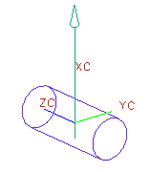J(moment of inertia) = 1/4 m*b^2 + 1/12 m*L^2
In our case the cylinder is not rotating around the axis in the middle of it, but around the axis which is a tangent on surface of this cylinder, so I think that the desired moment of inertia for our case considering Steiner's rule is:
J = m*b^2 + 1/4 m*b^2 + 1/12 m*L^2

Is my thinking correct?

Homework Helper
Gold Member
I think nothing changes except the moment of inertia.
Are the moment arms of the forces affected by b?
In our case the cylinder is not rotating around the axis in the middle of it, but around the axis which is a tangent on surface of this cylinder, so I think that the desired moment of inertia for our case considering Steiner's rule is:
J = m*b^2 + 1/4 m*b^2 + 1/12 m*L^2
OK (for small φ)

Last edited:
•srecko97
srecko97
Are the moment arms of the forces affected by b?
OK (for small φ)

Yep I forgot to consider that. The moment arms gets longer considering Pitagora's Theorem. arm^2= former arm^2 + b^2, right?

Homework Helper
Gold Member
Yep I forgot to consider that. The moment arms gets longer considering Pitagora's Theorem. arm^2= former arm^2 + b^2, right?
Does this give the correct moment arm for each side when φ = 0? Does the moment arm on the left side and the moment arm on the right side both get longer due to b?

Homework Helper
Gold Member
Yep I forgot to consider that. The moment arms gets longer considering Pitagora's Theorem. arm^2= former arm^2 + b^2, right?
No, that's not a good guess. Draw a diagram.

srecko97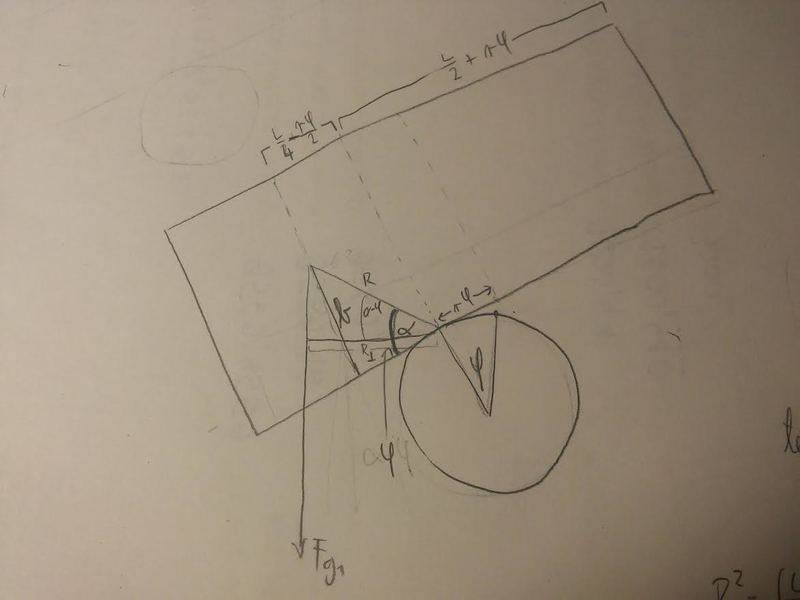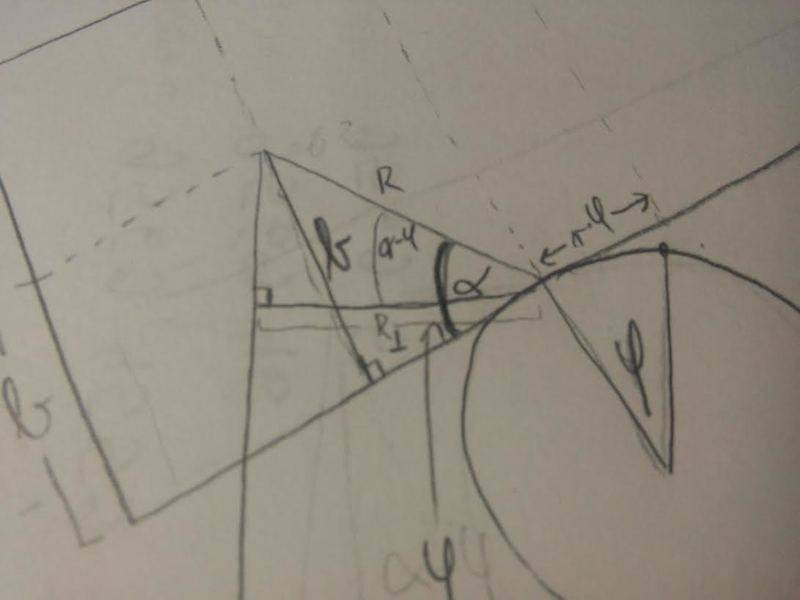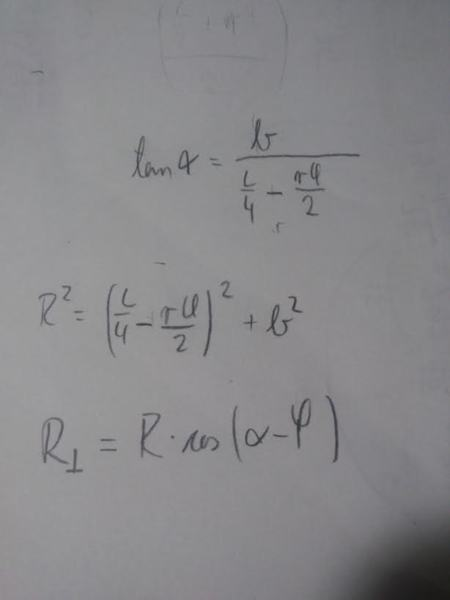I think it gets very very very very complicated if I consider the change of length of the momentum arm

srecko97
I do not have any data about b, so I cannot caluclate numbers for alpha or R, so it would get more difficult if I wanted to calculate the omega without numbers.

srecko97
Homework Helper
Gold Member
You are interested in how much the moment arm changes as you add the effect of b. Your R does not represent the change in the moment arm. Think about where the force on the left side acts before taking into account b and then after taking into account b.

srecko97
The force acts in the center of mass of the left part

srecko97
Honestly I do not understand clearly what you want to tell me. Can you help me please with some equations or sketches?

Homework Helper
Gold Member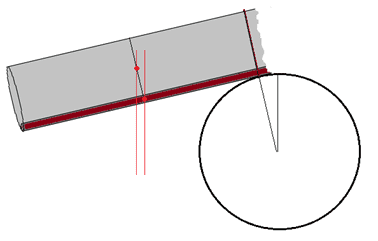srecko97
On the left the moment arm gets longer, but on the right it gets shorter

Homework Helper
Gold Member
On the left the moment arm gets longer, but on the right it gets shorter
Yes, that's true.

Homework Helper
Gold Member
I do not have any data about b, so I cannot caluclate numbers for alpha or R, so it would get more difficult if I wanted to calculate the omega without numbers.
Well, you won't get a numeric answer, but you should get a function of b that you can investigate.
Some labels for points:
A = Bottom left corner of the rod
A' = Top left corner
B = Point of contact.
B' = Point on top edge nearest B (so AB=A'B')
C = Mass centre of left hand portion (i.e. from AA' to BB')
D = Point on AB nearest to C
You already know the horizontal distance from B to D. You now want the horizontal distance from D to C to add to that. Can you see how to get that?

•srecko97
srecko97
the new moment arm is for b*tanφ longer?

Homework Helper
Gold Member
the new moment arm is for b*tanφ longer?
Apparently, this came from a triangle. Can you describe the triangle?

srecko97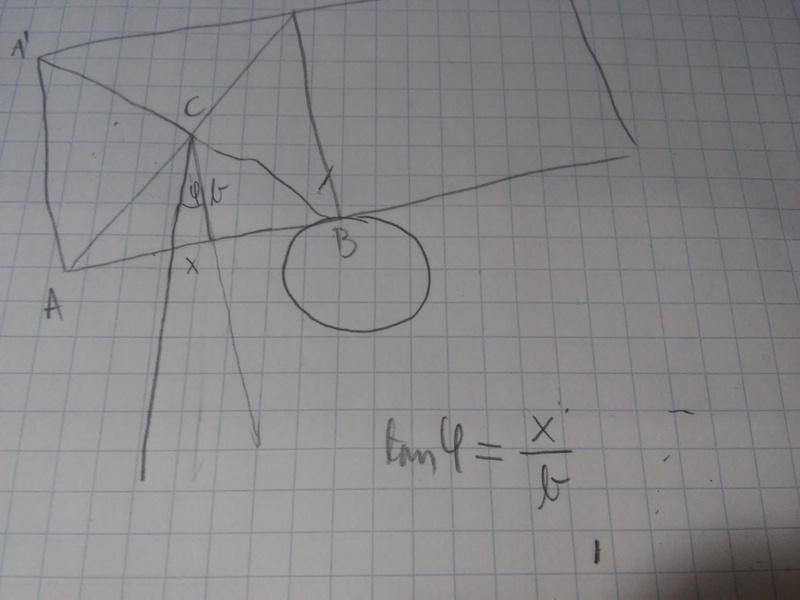Homework Helper
Gold Member
OK, We are probably using the term "moment arm" differently. I think of it as the perpendicular distance from the axis of rotation to the line of action of the force. You might be thinking of it as just the distance from the axis to the point of application of the force or something else?

srecko97
Ok, I know my previous attempt was wrong. You could think that I am just guessing the right equation, but no! Now I think that my answer is correct. The moment arm gets longer for b*sinφ, ok?

Homework Helper
Gold Member
The moment arm gets longer for b*sinφ, ok?
Yes, good.

srecko97
On the other side it gets shorter for b*sinφ

Homework Helper
Gold Member
On the other side it gets shorter for b*sinφ
Yes.

srecko97
But when talking about small φ that has no sense

Homework Helper
Gold Member
But when talking about small φ that has no sense
Why is that?

srecko97
sin φ ≈ 0 for small φ

Homework Helper
Gold Member
sin φ ≈ 0 for small φ
That's going too far in the approximation.